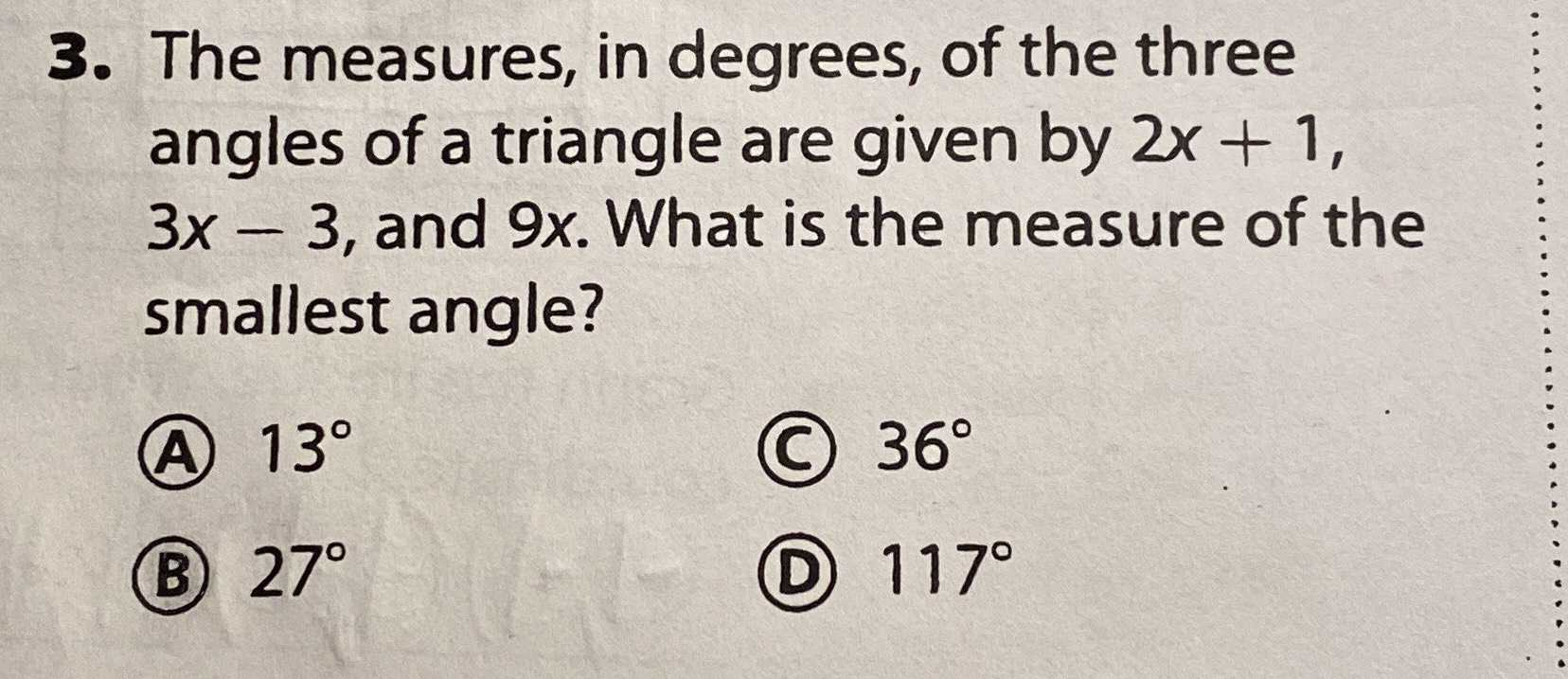### Still have math questions?

Trigonometry
QuestionThe measures, in degrees, of the three angles of a triangle are given by $$2 x + 1$$ , $$3 x - 3$$ , and $$9 x$$ . What is the measure of the smallest angle?

(A) $$13 ^ { \circ }$$ (C) $$36 ^ { \circ }$$

(B) $$27 ^ { \circ }$$ (D) $$117 ^ { \circ }$$